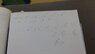# Yr 10 Indices problem

Darkmisc
Homework Statement:
Write 2^(-m) x 3^(-m) x 6^(2m) x 3^(2m) x 2^(2m) as a power of 6.
Relevant Equations:
Write 2^(-m) x 3^(-m) x 6^(2m) x 3^(2m) x 2^(2m) as a power of 6.
Hi everyone

Could someone please help me with a yr 10 maths problem? It's for my niece. I've done 2nd yr uni maths and can't seem to solve it.

Write 2^(-m) x 3^(-m) x 6^(2m) x 3^(2m) x 2^(2m) as a power of 6.

I've attached my attempt in the file. I get stuck at the point where I need to get rid of the base 2 and base 3 terms.

The answer is 6^(3m), but I don't know how to get there.

Thanks

#### Attachments

•WIN_20210204_20_36_28_Pro.jpg
40.3 KB · Views: 53

•